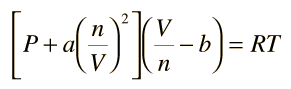# van der Waals Equation of State

The ideal gas law treats the molecules of a gas as point particles with perfectly elastic collisions. This works well for dilute gases in many experimental circumstances. But gas molecules are not point masses, and there are circumstances where the properties of the molecules have an experimentally measurable effect. A modification of the ideal gas law was proposed by Johannes D. van der Waals in 1873 to take into account molecular size and molecular interaction forces. It is usually referred to as the van der Waals equation of state.The constants a and b have positive values and are characteristic of the individual gas. The van der Waals equation of state approaches the ideal gas law PV=nRT as the values of these constants approach zero. The constant a provides a correction for the intermolecular forces. Constant b is a correction for finite molecular size and its value is the volume of one mole of the atoms or molecules.

 van der Waals Coefficients Gas a (Pa m6) b(m3/mol) Helium 3.46 x 10-3 23.71 x 10-6 Neon 2.12 x 10-2 17.10 x 10-6 Hydrogen 2.45 x 10-2 26.61 x 10-6 Carbon dioxide 3.96 x 10-1 42.69 x 10-6 Water vapor 5.47 x 10-1 30.52 x 10-6
Data from Fishbane, et al.

Since the constant b is an indication of molecular volume, it could be used to estimate the radius of an atom or molecule, modeled as a sphere. Fishbane et al. give the value of b for nitrogen gas as 39.4 x 10-6 m3/mol. This leads to the following estimate of radius:In the periodic table is found an atomic radius of 0.075 nm for nitrogen, so the above estimate for a nitrogen molecule is plausible.

With this "spherical molecule" assumption, a value b = x 10-6 m3/mol
corresponds to a molecular radius r = nm.

With the other parameter a = Pa m6 one can calculate the difference between the van der Waals equation of state and the ideal gas law.

In order to do a numerical exploration of the gas behavior, specify the pressure, volume and temperature. The number of moles will be calculated from the ideal gas law, and then the value PV/nT for the van der Waals equation of state will be calculated for comparison to the gas constant R.

For state variables
P = kPa = atmospheres
V = m3
T = K

the number of moles of an ideal gas would be n =

For the van der Waals equation of state this would give
PV/nT = J/mol K compared to the gas constant R = 8.3145 J/mol K.

The plot below (after Fishbane, et al.) shows significant departure from the ideal gas law, about 3% in 20 atmospheres pressure for oxygen. The departure is measurable even at one atmosphere pressure, but not large. The fact that all gases extrapolate to the same value is the basis for the definition of the Kelvin temperature scale in terms of that limiting value.Index

Gas law concepts

Kinetic theory concepts

Reference
Fishbane, Gasiorowicz, Thorton
Sec. 19-3

 HyperPhysics***** Thermodynamics R Nave
Go Back